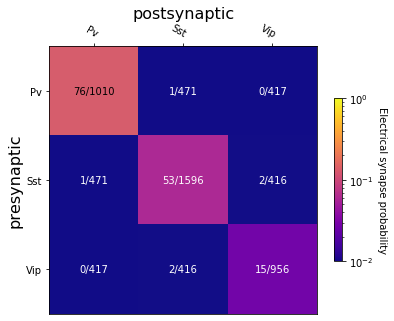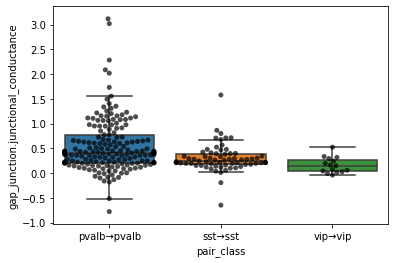# Exploring Electrical Synapses¶

In this notebook we use the Allen Institute synaptic physiology dataset to explore electrical synapses between inihibitory subclasses

For an introduction to the Jupyter Notebook interface, try Codeacademy: How To Use Jupyter Notebooks or Jupyter Notebook Quick Start Guide.

In :
from aisynphys.database import SynphysDatabase
from aisynphys.cell_class import CellClass, classify_cells, classify_pairs
from aisynphys.connectivity import measure_connectivity

c:\users\stephanies\appdata\local\continuum\miniconda3\envs\py3\lib\site-packages\statsmodels\tools\_testing.py:19: FutureWarning: pandas.util.testing is deprecated. Use the functions in the public API at pandas.testing instead.
import pandas.util.testing as tm

In [ ]:
# Download and cache the sqlite file for the requested database
#   (for available versions, see SynphysDatabase.list_versions)


Electrical synapses (sometimes referred to as gap junctions) primarily exist between like inhibitory subclasses. Here we will explore the electrical connectivity of innhibitory cells and strength of electrical synapses as measured by junctional conductance.

Let's begin by defining our inhibitory cell subclasses:

In :
inhib_subclasses = {
'pv': CellClass(cre_type='pvalb', name='pv'),
'sst': CellClass(cre_type='sst', name='sst'),
'vip': CellClass(cre_type='vip', name='vip'),
}


We'll now load all of our mouse pairs to measure the rate of electrical connectivity among these subclasses

In :
mouse_pairs = db.pair_query(experiment_type='standard multipatch', species='mouse').all()
print(len(mouse_pairs))

83006


First we group all of the cells by their subclass, then create all of the possible pair-group combinations, and finally measure the connectivity between them. Note that the measure_connectiviy method measures both chemical and electrical connectivity, but that here we use a shorter sigma to fit the distance-adjusted model. See the Connectivity Adjustment notebook as well as our paper [] for more details.

In :
# Group all cells by selected subclasses
cell_groups = classify_cells(inhib_subclasses.values(), pairs=mouse_pairs)

# Group pairs into (pre_class, post_class) groups
pair_groups = classify_pairs(mouse_pairs, cell_groups)

# analyze matrix elements
results = measure_connectivity(pair_groups, sigma=75e-6, dist_measure='lateral_distance')


Now we can plot the results as a matrix

In :
import matplotlib.colors, matplotlib.cm
import matplotlib.pyplot as plt
from aisynphys.ui.notebook import show_connectivity_matrix
%matplotlib inline

# define a colormap and log normalization used to color the heatmap
norm = matplotlib.colors.LogNorm(vmin=0.01, vmax=1.0, clip=True)
cmap = matplotlib.cm.get_cmap('plasma')

# define the display labels to use for each cell subclass:
class_labels = {
'pv': 'Pv',
'sst':  'Sst',
'vip': 'Vip',
}

# create a figure/axes to draw on
fig, ax = plt.subplots(figsize=(6, 6))

# finally, draw the colormap using the provided function:
im, cbar, labels = show_connectivity_matrix(
ax=ax,
results=results,
pre_cell_classes=inhib_subclasses.values(),
post_cell_classes=inhib_subclasses.values(),
class_labels=class_labels,
cmap=cmap,
norm=norm,
ctype='electrical', # here we change the ctype from the default "chemical" to "electrical"
cbarlabel='Electrical synapse probability',
)We measure the strength of electrical synapses using junctional conductance. This can be extracted from the gap_junction table along with other parameters such as coupling coefficient and cross-correlation values during the stimulus pulse and during baseline periods.

We can again query the database for mouse pairs but this time filter just for electrical connections between like subclasses

In :
# query once for each postsynaptic type, building up a Pandas dataframe

pairs = None
for name, subclass in inhib_subclasses.items():
pair_query = db.pair_query(
experiment_type='mouse',
pre_class=subclass,
post_class=subclass,
electrical=True,
)
# here we join the GapJunction table so that we can append the junctional_conductance column
pair_query = pair_query.outerjoin(db.GapJunction, db.GapJunction.pair_id==db.Pair.id)

df = pair_query.dataframe()
df['pair_class'] = '%s→%s' % (subclass, subclass)
if pairs is None:
pairs = df
else:
pairs = pairs.append(df)
pairs['gap_junction.junctional_conductance'] *= 1e9 # we typcially think of conductance on the scale of nano-Siemens (nS)

Out:
pair.id pair.experiment_id pair.pre_cell_id pair.post_cell_id pair.has_synapse pair.has_polysynapse pair.has_electrical pair.crosstalk_artifact pair.n_ex_test_spikes pair.n_in_test_spikes pair.distance pair.lateral_distance pair.vertical_distance pair.reciprocal_id pair.meta gap_junction.junctional_conductance pair_class
0 52178 1539 9086 9087 False False True NaN 418 300 0.000092 0.000027 0.000078 52189 None 1.156760 pvalb→pvalb
1 52189 1539 9087 9086 False False True NaN 417 299 0.000092 0.000027 0.000078 52178 None 1.494854 pvalb→pvalb
2 113767 3981 21393 21392 True False True NaN 420 348 0.000084 NaN NaN 113765 None 0.245593 pvalb→pvalb
3 123654 5109 24793 24795 False False True NaN 507 308 0.000101 NaN NaN 123661 None 0.665745 pvalb→pvalb
4 123679 5112 24801 24802 False False True NaN 1599 1284 0.000062 NaN NaN 123682 None 0.776390 pvalb→pvalb

From here we can plot the junctional conductance using the seaborn library

In :
import seaborn as sns

sns.boxplot(data=pairs, x='pair_class', y='gap_junction.junctional_conductance', fliersize=0)
sns.swarmplot(data=pairs, x='pair_class', y='gap_junction.junctional_conductance', color='k', edgecolor='k', alpha=0.7)

c:\users\stephanies\appdata\local\continuum\miniconda3\envs\py3\lib\site-packages\seaborn\categorical.py:1296: UserWarning: 8.8% of the points cannot be placed; you may want to decrease the size of the markers or use stripplot.
warnings.warn(msg, UserWarning)
c:\users\stephanies\appdata\local\continuum\miniconda3\envs\py3\lib\site-packages\seaborn\categorical.py:1296: UserWarning: 6.0% of the points cannot be placed; you may want to decrease the size of the markers or use stripplot.
warnings.warn(msg, UserWarning)

Out:
<matplotlib.axes._subplots.AxesSubplot at 0x2324db1afc8>Here we can see that electrical connections between Pvalb cells can be stronger than those between Sst or Vip cells

In [ ]: